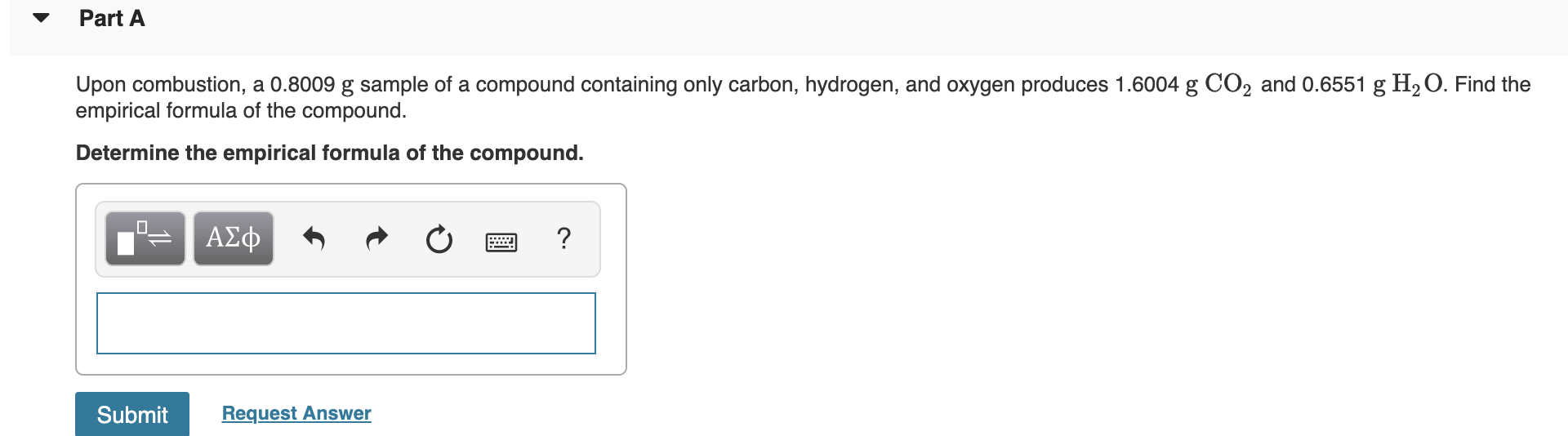Part AUpon combustion, a 0.8009 g sample of a compound containing only carbon, hydrogen, and oxygen produces 1.6004 g CO2 and 0.6551 g H2 O. Find theempirical formula of the compound.Determine the empirical formula of the compound.ΑΣφ?SubmitRequest Answer

Questionhelp_outlineImage TranscriptionclosePart A Upon combustion, a 0.8009 g sample of a compound containing only carbon, hydrogen, and oxygen produces 1.6004 g CO2 and 0.6551 g H2 O. Find the empirical formula of the compound. Determine the empirical formula of the compound. ΑΣφ ? Submit Request Answer fullscreen
Step 1

The combustion reactions are a type of chemical reactions where hydrocarbons react with oxygen and result in the production of water and carbon dioxide.

Step 2

The number of moles of a given chemical species is defined as the ratio of mass to the molar mass of the substance. The mathematical formula is as shown below:

Step 3

The empirical formula of a given compound refers to the simples...

Want to see the full answer?

See Solution

Want to see this answer and more?

Our solutions are written by experts, many with advanced degrees, and available 24/7

See Solution
Tagged in

Inorganic Chemistry